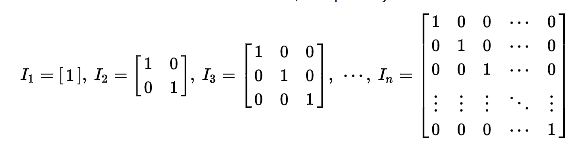Solution 1

``````def identity_matrix(size):
matrix = []
for i in range(size):
row = []
for j in range(size):
if i == j:
row.append(1)
else:
row.append(0)
matrix.append(row)
return matrix
``````

# Identity Matrix

An identity matrix is defined in the following way:Basically, all the elements in the diagonal are ones (`1`s) and the rest are zeros (`0`s).

An identity matrix has a `size` associated. For example, this is an identity matrix of size 3:

``````1 0 0
0 1 0
0 0 1
``````

And this is a matrix of size 5:

``````1 0 0 0 0
0 1 0 0 0
0 0 1 0 0
0 0 0 1 0
0 0 0 0 1
``````

A matrix is represented, Pythonically, with a "list of lists". Example:

``````# size 3
size_3_matrix = [
[1, 0, 0],
[0, 1, 0],
[0, 0, 1],
]
# size 5
size_5_matrix = [
[1, 0, 0, 0, 0],
[0, 1, 0, 0, 0],
[0, 0, 1, 0, 0],
[0, 0, 0, 1, 0],
[0, 0, 0, 0, 1],
]
``````

Write a function `identity_matrix` that receives a `size` parameter and builds an identity matrix using "lists of lists".

### Test Cases

test size 5 identity matrix -

``````size_5_matrix = [
[1, 0, 0, 0, 0],
[0, 1, 0, 0, 0],
[0, 0, 1, 0, 0],
[0, 0, 0, 1, 0],
[0, 0, 0, 0, 1],
]

def test_size_5_identity_matrix():
assert identity_matrix(5) == size_5_matrix
``````

test size 2 identity matrix -

``````size_2_matrix = [
[1, 0],
[0, 1],
]

def test_size_2_identity_matrix():
assert identity_matrix(2) == size_2_matrix
``````

test size 3 identity matrix -

``````size_3_matrix = [
[1, 0, 0],
[0, 1, 0],
[0, 0, 1],
]

def test_size_3_identity_matrix():
assert identity_matrix(3) == size_3_matrix
``````
def identity_matrix(size): pass